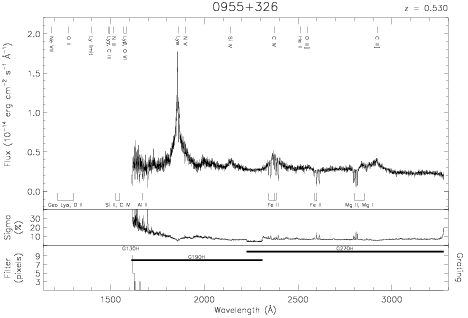Download dataset
TELESCOP= 'HST     '
INSTRUME= 'FOS     '
RADECSYS= 'FK5     '
EQUINOX =               2000.0
DATE-OBS= '1992-12-25T22:54:53'
RA_PNT  =       149.5875144316
DEC_PNT =        32.4005788337
PA_PNT  =        22.0991778859
OBJECT  = '0955+326'
ROOTNM01= 'Y10Q0103T'
GRNDMD01= 'RAPID-READOUT'
DETECT01= 'AMBER   '
APERID01= 'C-2     '
FGWAID01= 'H27     '
ROOTNM02= 'Y10Q0104T'
GRNDMD02= 'RAPID-READOUT'
DETECT02= 'AMBER   '
APERID02= 'C-2     '
FGWAID02= 'H19     '
ROOTNM03= 'Y10Q0105T'
GRNDMD03= 'RAPID-READOUT'
DETECT03= 'AMBER   '
APERID03= 'C-2     '
FGWAID03= 'H19     '
ROOTNM04= 'Y1HI0802T'
GRNDMD04= 'SPECTROSCOPY'
DETECT04= 'BLUE    '
APERID04= 'A-1     '
FGWAID04= 'H13     '